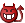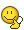## Recommended Posts

Hi everyone,

I have been trying to practice calculus for the benfit of my quantum mechanics studies and I am stuck with a problem so thought you people might have some advice to give.Here goes;

$\frac{d sin\theta}{d(\theta)cos(\theta)} sin(\theta)sin(\phi)$

Now we have to operate the $\frac{d sin(\theta)}{d(\theta)cos(\theta)}$ onto function $sin(\theta)sin(\phi)$.

The operator can expressed into $cos^2(\theta)-sin^2(\theta)$ after diffentiation. Now my question is that can I simply multiply this by $sin(\theta)sin(\phi)$ or will this be wrong?

It would have been much easier if the expression were $\frac{d}{d(\theta)} sin(\theta)sin(\phi)$ i.e. to diffentiatie the function?!

I'll be grateful for your help!

##### Share on other sites

Hi everyone,

I have been trying to practice calculus for the benfit of my quantum mechanics studies and I am stuck with a problem so thought you people might have some advice to give.Here goes;

$\frac{d sin\theta}{d(\theta)cos(\theta)} sin(\theta)sin(\phi)$

Now we have to operate the $\frac{d sin(\theta)}{d(\theta)cos(\theta)}$ onto function $sin(\theta)sin(\phi)$.

The operator can expressed into $cos^2(\theta)-sin^2(\theta)$ after diffentiation. Now my question is that can I simply multiply this by $sin(\theta)sin(\phi)$ or will this be wrong?

It would have been much easier if the expression were $\frac{d}{d(\theta)} sin(\theta)sin(\phi)$ i.e. to diffentiatie the function?!

I'll be grateful for your help!

no you cannot simply multiply sin(theta) by sin(phi) to simplify, they are separate unknown variables.

the expression could not be $\frac{d}{d(\theta)} sin(\theta)sin(\phi)$ your sin(theta) should be sin squared(theta).

##### Share on other sites

well this is why I am slighty confused. The question goes to operate wavefunction by an operator to check if the wavefunction is an eigenfunction of the operator.

The operator is $U = \frac{d sin(\theta)}{d(\theta)cos(\theta)}$ and the function is $f(\theta, \phi) = sin(\theta)sin(\phi)$

Now I know that I will have to use the product rule , but I dont know how, becuase the operator is very strange. Its like its saying that diffentiate the function f by $\frac{d sin(\theta)}{d(\theta)cos(\theta)}$

Maybe i havent understood the question properly?

##### Share on other sites

I just gave my two bits on basis of what I know from algebra/trig. I want to help you answer you question but my math skills are limited lol. Someone more qualified that me please help this man!

##### Share on other sites

For a start: I think U is not an operator but a terrible mess. Do you know what U means? I don't. Is it really the operator as it is given in the question/book or did you rearrange some letters?

E.g. if U was $\frac{\sin \theta}{\cos \theta} \frac{d}{d\theta}$ then f would obviously be an eigenfuntion.

##### Share on other sites

For a start: I think U is not an operator but a terrible mess. Do you know what U means? I don't. Is it really the operator as it is given in the question/book or did you rearrange some letters?

E.g. if U was $\frac{\sin \theta}{\cos \theta} \frac{d}{d\theta}$ then f would obviously be an eigenfuntion.

You know what atheist, you got it right. The way the question was written down I didnt understand it. U is exactly as you suggested;

$\frac{\sin \theta}{\cos \theta} \frac{d}{d\theta}$

but I am not sure yet if it is an eigenfunction, so I will do the calculations but I know how to do that. Thanks a lot.

Merged post follows:

Consecutive posts merged

just one more question. Its the double derivative question;

$\frac {d^2}{dx^2} e^\frac{-x^2}{2}$

and this is what I did, frst taking the first derivate:

$\frac {d}{dx} e^\frac{-x^2}{2} = \frac{-1}{2}e^\frac{-x^2}{2}$

Now taking the second derivative;

$\frac {d^2}{dx^2} (\frac{-1}{2}e^\frac{-x^2}{2})=\frac{-x}{4} e^\frac{-x^2}{2}$

could anybody confirm id this is right or not? any help most appreciated!

##### Share on other sites

and this is what I did, frst taking the first derivate:

$\frac {d}{dx} e^\frac{-x^2}{2} = \frac{-1}{2}e^\frac{-x^2}{2}$

chain rule $\frac{df(g(x))}{dx}= \frac{df}{dg}\frac{dg}{dx}$

letting $f(y)=e^y$ and $g(x)=-x^2$, then $\frac{dg}{dx}$ is clearly going to be be proportional to x to the 1st power. This is definately missing from your expression above.

## Create an account

Register a new account